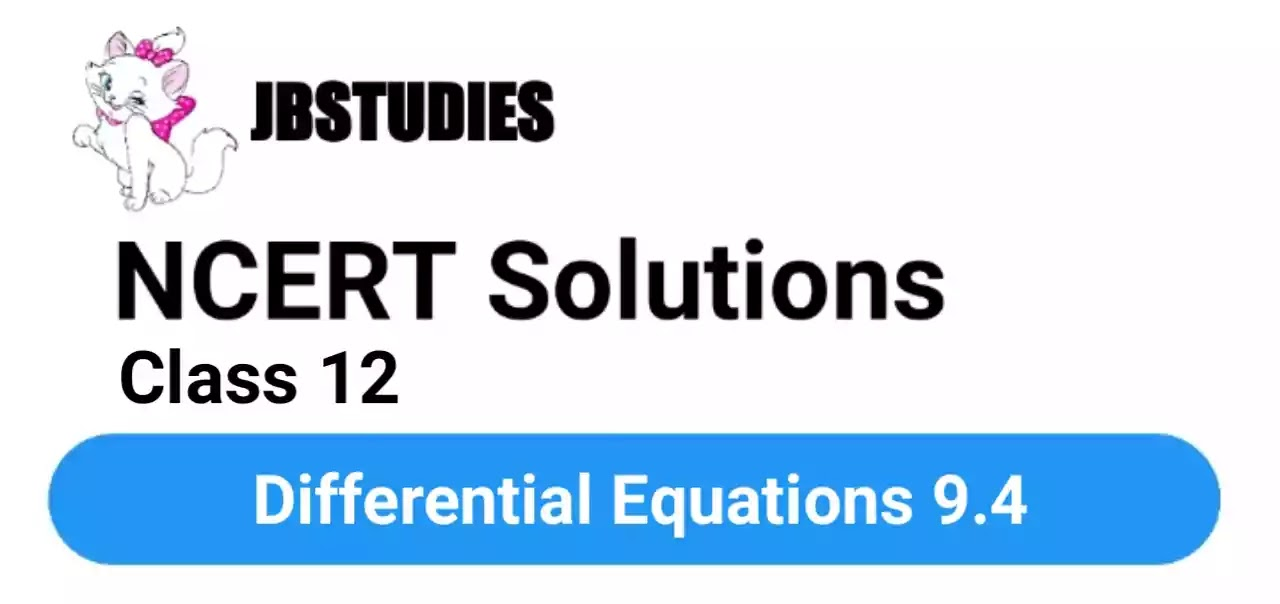# NCERT Solutions Class 12 Maths Chapter-9 (Determinants)Exercise 9.4

NCERT Solutions Class 12 Maths from class 12th Students will get the answers of Chapter-9 (Determinants)Exercise 9.4 This chapter will help you to learn the basics and you should expect at least one question in your exam from this chapter.
We have given the answers of all the questions of NCERT Board Mathematics Textbook in very easy language, which will be very easy for the students to understand and remember so that you can pass with good marks in your examination.### Exercise 9.4

Q1.

Q2.

Q3.

Answer.  $\begin{array}{rl}& \int \frac{dy}{1-y}=\int dx\\ & ⇒\mathrm{log}\left(1-y\right)=x+\mathrm{log}\mathrm{C}\\ & ⇒-\mathrm{log}C-\mathrm{log}\left(1-y\right)=x\\ & ⇒\mathrm{log}\mathrm{C}\left(1-y\right)=-x\\ & ⇒\mathrm{C}\left(1-y\right)={e}^{-x}\end{array}$

Q4.

Q5.

Q6.